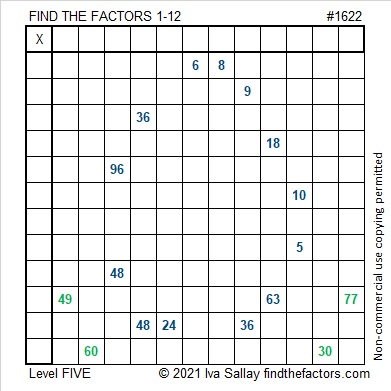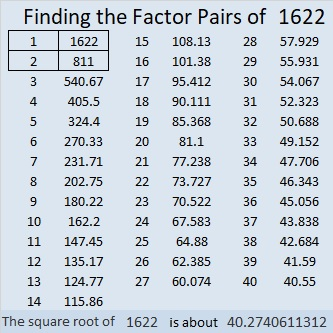Contents

### Today’s Puzzle:

These somewhat tricky level-5 puzzles are probably better suited for middle school and up than younger kids. Use logic on every step and you should be able to find its unique solution.Here are some Easter egg puzzles I saw on Twitter. Some are perfect for the littles and others are for older kids. Easter egg hunts can be fun for anyone of any age.

### Factors of 1622:

• 1622 is a composite number.
• Prime factorization: 1622 = 2 × 811.
• 1622 has no exponents greater than 1 in its prime factorization, so √1622 cannot be simplified.
• The exponents in the prime factorization are 1 and 1. Adding one to each exponent and multiplying we get (1 + 1)(1 + 1) = 2 × 2 = 4. Therefore 1622 has exactly 4 factors.
• The factors of 1622 are outlined with their factor pair partners in the graphic below.### More about the Number 1622:

1622 is the sum of four consecutive numbers:
409 + 410 + 411 + 412 = 1622.

This site uses Akismet to reduce spam. Learn how your comment data is processed.